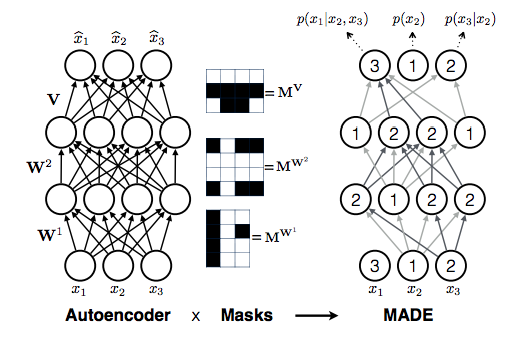April 15, 2019

homepage
language Python
size (curr.) 87 kB
stars (curr.) 382
created 2018-04-22

This code is an implementation of “Masked AutoEncoder for Density Estimation” by Germain et al., 2015. The core idea is that you can turn an auto-encoder into an autoregressive density model just by appropriately masking the connections in the MLP, ordering the input dimensions in some way and making sure that all outputs only depend on inputs earlier in the list. Like other autoregressive models (char-rnn, pixel cnns, etc), evaluating the likelihood is very cheap (a single forward pass), but sampling is linear in the number of dimensions.The authors of the paper also published code here, but it’s a bit wordy, sprawling and in Theano. Hence my own shot at it with only ~150 lines of code and PyTorch <3.

## examples

First we download the binarized mnist dataset. Then we can reproduce the first point on the plot of Figure 2 by training a 1-layer MLP of 500 units with only a single mask, and using a single fixed (but random) ordering as so:

``````python run.py --data-path binarized_mnist.npz -q 500
``````

which converges at binary cross entropy loss of `94.5`, as shown in the paper. We can then simultaneously train a larger model ensemble (with weight sharing in the one MLP) and average over all of the models at test time. For instance, we can use 10 orderings (`-n 10`) and also average over the 10 at inference time (`-s 10`):

``````python run.py --data-path binarized_mnist.npz -q 500 -n 10 -s 10
``````

which gives a much better test loss of `79.3`, but at the cost of multiple forward passes. I was not able to reproduce single-forward-pass gains that the paper alludes to when training with multiple masks, might be doing something wrong.

## usage

The core class is `MADE`, found in `made.py`. It inherits from PyTorch `nn.Module` so you can “slot it into” larger architectures quite easily. To instantiate MADE on 1D inputs of MNIST digits for example (which have `28*28` pixels), using one hidden layer of 500 neurons, and using a single but random ordering we would do:

``````model = MADE(28*28, , 28*28, num_masks=1, natural_ordering=False)
``````

The reason we plug the size of the output (3rd argument) into MADE is that one might want to use relatively complicated output distributions, for example a gaussian distribution would normally be parameterized by a mean and a standard deviation for each dimension, or you could bin the output range into buckets and output logprobs for a softmax, or mixture parameters, etc. In the simplest example in this code we use binary predictions, where are only parameterized by one number, hence the number of the input dimensions happens to equal the number of outputs.